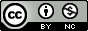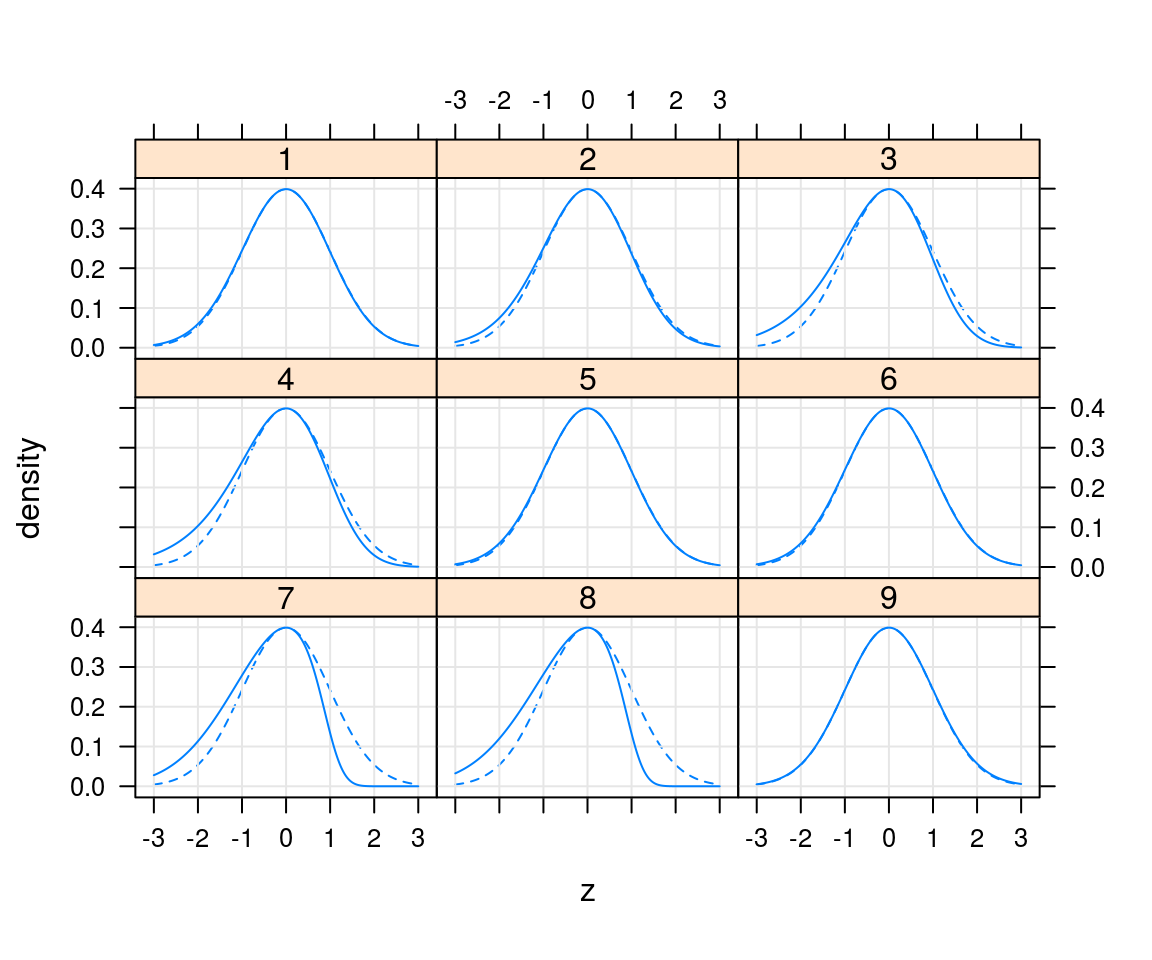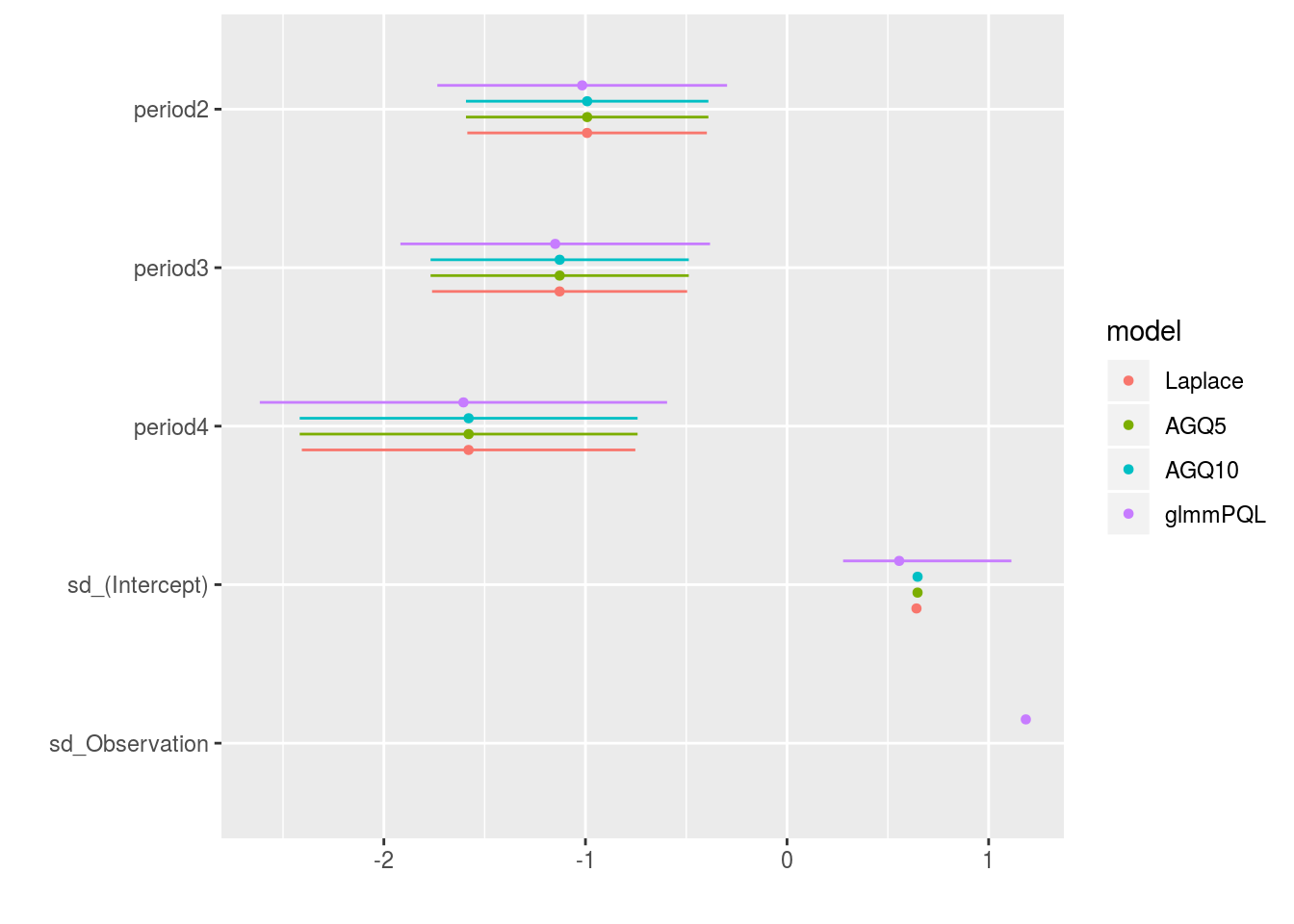Licensed under the Creative Commons attribution-noncommercial license. Please share & remix noncommercially, mentioning its origin.

library(lme4)
library(broom)
library(broom.mixed)
library(dotwhisker)

## Overview

Most aspects of GLMMs are carried over from LMMs (random effects) and GLMs (families and links).

## integration methods

You do have to decide on an approximation method.

• penalized quasi-likelihood
• fastest/least accurate
• uses quasi-likelihood (no AIC etc.?)
• Laplace approximation
• fast, flexible compromise
• slowest
• most accurate
• most limited

In lme4, use the nAGQ= argument; nAGQ=1 (default) corresponds to Laplace approximation

## integration rules

• it only really matters in relatively extreme cases (small numbers of binary obs per group)
• use the most accurate method that’s available and feasible

## Laplace-approximation diagnostics

library(lattice)
aspect <- 0.6
xlab <- "z"; ylab <- "density"; type <- c("g","l"); scaled <- FALSE
print(xyplot(y ~ zvals|id, data=mm,
type=type, aspect=aspect,
xlab=xlab,ylab=ylab,
as.table=TRUE,
panel=function(x,y,...){
if (!scaled) {
panel.lines(x, dnorm(x), lty=2)
} else {
panel.abline(h=1, lty=2)
}
panel.xyplot(x,y,...)
}))## comparing integration methods

g1 <- glmer(incidence/size ~ period + (1|herd),
family=binomial,
data=cbpp,
weights=size)
g2 <- update(g1,nAGQ=5)
g3 <- update(g1,nAGQ=10)
g4 <- MASS:::glmmPQL(incidence/size ~ period,
random = ~1|herd,
data=cbpp,
family=binomial,
weights=size)
## iteration 1
## iteration 2
## iteration 3
## iteration 4
dwplot(list(Laplace=g1,AGQ5=g2,AGQ10=g3,glmmPQL=g4))## overdispersion

• reminder: “too much” variance
• only applies to families with estimated variance:
e.g. Poisson, binomial ($$N>1$$)
• sum^2 of Pearson residuals / residual degrees of freedom
• e.g. aods3::gof()
• observation-level random effects

## dealing with overdispersion

• quasi-likelihood
• observation-level random effects (Elston et al. 2001)
• overdispersed distributions
(negative binomial, beta-binomial, etc.)
glmmTMB/brms (Brooks et al. 2017)

## other diagnostics

• assumption of Normal residuals may not be very good
• simulated residuals (DHARMa)

## zero-inflation

• glmmTMB etc.
• Owls example

## Complete separation

• penalized regression